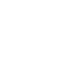Menu# Maximum measurements and weights

### COURIER PARCELS

DPD calculates the transport cost of consignments up to 31.5 kg (0.126 cbm) on the basis of actual weight (kg). Maximum permissible dimensions for one parcel: the sum of the longest side and the bottom girth is 3.0 m, while the longest side cannot exceed 1.75 m. Maximum dimensions are necessary to ensure the fast, safe and smooth handling of parcels on parcel sorting lines at distribution centres.

Maximum dimensions of a DPD parcel:

• Length: maximum 175 cm (longest side);

• Sum of longest side and bottom girth: 3.0 m [2 x (height + width)+longest side];

• Parcel unit weight: 31.5 kg

• Attention: parcels weighing more than 31.5 kg must be placed on a pallet!

Miminum size: A5

# Girth and Volumetric Weight Calculator

Height (cm)Width (cm)Length (cm)

The combined dimensions of your parcel are:

cm

The volumetric weigth of your parcel:

KG

NOTE: This is a computed value which was not measured with calibrated scales - please see the note in the first paragraph.

Volumetric weight

Indicator calculated using the dimensions of the Consignment, helping to estimate how much room the Consignment will take in the means of transportation. Volumetric Weight is calculated according to the following formula: length (cm) × width (cm) × height (cm) / 4,000 (i.e., 1 m3=250 kg). If the Actual Weight and Volumetric Weight of the Consignment are different, DPD has the right to proceed based on the bigger of the two for providing the Service or determining the price.

XS parcel: height 8 cm, width 18 cm, depth 61 cm
S parcel: height 8 cm, width 43 cm, depth 61 cm
M parcel: height 17 cm, width 43 cm, depth 61 cm
L parcel: height 36 cm, width 43 cm, depth 61 cm

Maximum weight in Baltics up to 31,5 kg, rest of Europe 20 kg (Portugal 10 kg).

Minimum size: A5The maximum permissible weight of one parcel sent with DPD is 31.5 kg (0.126 cbm). From 31.5 kg upwards, the transport price of the parcel is calculated on the basis of the estimated weight: the actual and volumetric weight of the consignment are compared and the higher of the two is considered. Parcels heavier than 31.5 kg must be placed on a pallet for transport.

Maximum dimensions of a consignment on a pallet:

Maximum length: 1.8 m (lowest estimated height of the parcel 1.0 m);

Weight: 31.6 kg – 700 kg.

Note: If you have more than one parcel and want to calculate the total volumetric weight, add the volumetric weight of the parcels together.